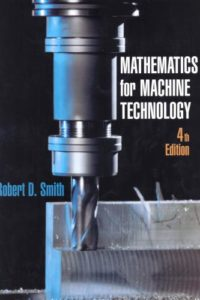اسم المؤلف
Robert D. Smith
التاريخ
9 يوليو 2018
المشاهدات
التقييمLoading...

Mathematics for Machine Technology
Fourth Edition
Robert D. Smith
CONTENTS
Preface ix
SECTION 1 Common Fractions, Decimal Fractions, and Percentage 1
Introduction to Common Fractions and Mixed Numbers
Addition of Common Fractions and Mixed Numbers
Subtraction of Common Fractions and Mixed Numbers
Multiplication of Common Fractions and Mixed Numbers
Division of Common Fractions and Mixed Numbers
Combined Operations of Common Fractions and
Mixed Numbers
Computing with a Calculator: Fractions and Mixed Numbers
Introduction to Decimal Fractions
Rounding Decimal Fractions and Equivalent Decimal and
Common Fractions
Addition and Subtraction of Decimal Fractions
Multiplication of Decimal Fractions
Division of Decimal Fractions
Powers
Roots
Table of Decimal Equivalents and Combined Operations of
Decimal Fractions
Computing with a Calculator: Decimals
Introduction to Percents
Basic Calculations of Percentages, Percents, and Rates
Percent Practical Applications
Achievement Review Section One
SECTION 2 Linear Measurement: English and Metric 95
UNIT 21 English and Metric Units of Measure
UNIT 22 Degree of Precision, Greatest Possible Error, Absolute Error,
and Relative Error
UNIT 23 Tolerance, Clearance, and Interference
UNIT 24 English and Metric Steel Rules
UNIT 25 English Vernier Calipers and Height Gages
UNIT 26 Metric Vernier Calipers and Height Gages
UNIT 27 English Micrometers
VVi MATHEMATICS FOR MACHINE TECHNOLOGY
UNIT 28 Metric Micrometers 141
UNIT 29 English and Metric Gage Blocks
UNIT 30 Achievement Review
Section Two
SECTION 3 Fundamentals of Algebra 155
UNIT 31 Symbolism
UNIT 32 Signed Numbers
UNIT 33 Algebraic Operations of Addition, Subtraction,
and Multiplication
UNIT 34 Algebraic Operations of Division, Powers, and Roots
UNIT 35 Introduction to Equations
UNIT 36 Solution of Equations by the Subtraction, Addition,
and Division Principles of Equality
UNIT 37 Solution of Equations by the Multiplication, Root,
and Power Principles of Equality
UNIT 38 Solution of Equations Consisting of Combined Operations and
Rearrangement of Formulas
UNIT 39 Ratio and Proportion
UNIT 40 Direct and Inverse Proportions
UNIT 41 Applications of Formulas to Cutting Speed, Revolutions
Per Minute, and Cutting Time
UNIT 42 Applications of Formulas to Spur Gears
UNIT 43 Achievement Review Section Three
SECTION 4 Fundamentals of Plane Geometry 258
UNIT 44 Introduction to Geometric Figures
UNIT 45 Protractors Simple and Vernier
UNIT 46 Angles
UNIT 47 Introduction to Triangles
UNIT 48 Geometric Principles for Triangles and Other Common
Polygons
UNIT 49 Introduction to Circles
UNIT 50 Arcs and Angles of Circles
UNIT 51 Fundamental Geometric Constructions
UNIT 52 Achievement Review Section Four
SECTION 5 Trigonometry 333
UNIT 53 Introduction to Trigonometric Functions
UNIT 54 Analysis of Trigonometric Functions
UNIT 55 Basic Calculations of Angles and Sides of Right Triangles
UNIT 56 Simple Practical Machine Applications
UNIT 57 Complex Practical Machine Applications 361
UNIT 58 The Cartesian Coordinate System
UNIT 59 Oblique Triangles: Law of Sines and Law of Cosines
UNIT 60 Achievement Review
Section Five
SECTION 6 Compound Angles 390
UNIT 61 Introduction to Compound Angles 390
UNIT 62 Drilling and Boring Compound-Angular Holes: Computing
Angles of Rotation and Tilt Using Given Lengths
UNIT 63 Drilling and Boring Compound-Angular Holes: Computing
Angles of Rotation and Tilt Using Given Angles
UNIT 64 Machining Compound-Angular Surfaces: Computing Angles
of Rotation and Tilt
UNIT 65 Computing Angles Made by the Intersection of Two
Angular Surfaces
UNIT 66 Computing Compound Angles on Cutting and Forming Tools . … 418
UNIT 67 Achievement Review Section Six
SECTION 7 Computer Numerical Control (CNC) 428
UNIT 68 Introduction to Computer Numerical Control (CNC) 428
UNIT 69 Control Systems, Absolute Positioning, Incremental
Positioning
UNIT 70 Binary Numeration System
UNIT 71 Achievement Review
Section Seven
Appendix 446